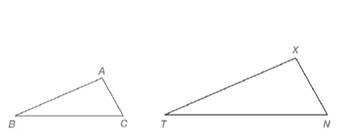Chapter 5.2, Problem 5EElementary Geometry For College St...

7th Edition
Alexander + 2 others
ISBN: 9781337614085

Solutions

Chapter
SectionElementary Geometry For College St...

7th Edition
Alexander + 2 others
ISBN: 9781337614085
Textbook Problem

In Exercises 5 and 6, refer to the drawing.a) Given that A ↔ X , B ↔ T , and C ↔ N , write a statement claiming that the triangles shown are similar.b) Given that A ↔ N , C ↔ X , and B ↔ T , write a statement claiming that the triangles shown are similar.Exercises 5, 6

To determine

a)

To write:

A statement claiming that the triangles shown are similar.

Explanation

Definition:

Similarity between Two polygons:

Two polygons are similar if and only if when all pairs of corresponding angles are congruent and all pairs of corresponding sides are proportional.

Given:

The triangles ABC and XTN are similar.

AX,BT,and CN.

Calculation:

According to the definition of similar polygons, the corresponding angles are congruent and corresponding sides are proportional

To determine

b)

To write:

A statement claiming that the triangles shown are similar.

Still sussing out bartleby?

Check out a sample textbook solution.

See a sample solution

The Solution to Your Study Problems

Bartleby provides explanations to thousands of textbook problems written by our experts, many with advanced degrees!

Get Started

Exponential Form Write the equation in exponential form. 23. log x = y

Precalculus: Mathematics for Calculus (Standalone Book)

In Exercises 914, evaluate the expression. 11. (3 4)2

Applied Calculus for the Managerial, Life, and Social Sciences: A Brief Approach

Solve the equations in Exercises 112 for x (mentally, if possible). 2x+4=1

Finite Mathematics and Applied Calculus (MindTap Course List)

Fill in each blank: 5lb3oz=oz

Elementary Technical Mathematics

In problems 9-18, simplify the expressions with all exponents positive. 18.

Mathematical Applications for the Management, Life, and Social Sciences

True or False: If F is conservative, then curl F = 0.

Study Guide for Stewart's Multivariable Calculus, 8th